Share

# NCERT solutions for Class 7 Maths chapter 15 - Visualising Solid Shapes [Latest edition]

Course
Textbook page

#### Chapters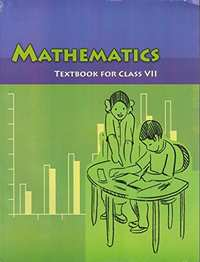## Chapter 15: Visualising Solid Shapes

Ex. 15.1Ex. 15.2Ex. 15.3Ex. 15.4

#### NCERT solutions for Class 7 Maths Chapter 15 Visualising Solid Shapes Exercise 15.1 [Pages 281 - 282]

Ex. 15.1 | Q 1.1 | Page 281

Identify the nets which can be used to make cubes (cut out copies of the nets and try it):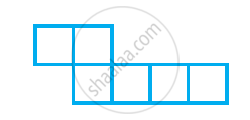Ex. 15.1 | Q 1.2 | Page 281

Identify the nets which can be used to make cubes (cut out copies of the nets and try it):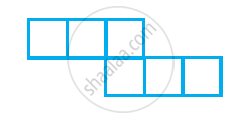Ex. 15.1 | Q 1.3 | Page 281

Identify the nets which can be used to make cubes (cut out copies of the nets and try it):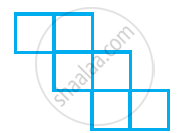Ex. 15.1 | Q 1.4 | Page 281

Identify the nets which can be used to make cubes (cut out copies of the nets and try it):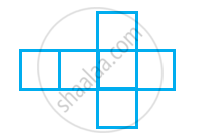Ex. 15.1 | Q 1.5 | Page 281

Identify the nets which can be used to make cubes (cut out copies of the nets and try it):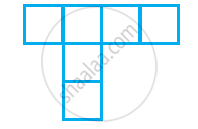Ex. 15.1 | Q 1.6 | Page 281

Identify the nets which can be used to make cubes (cut out copies of the nets and try it):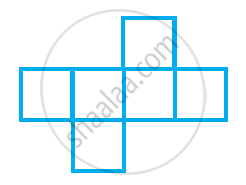Ex. 15.1 | Q 2 | Page 281

Dice are cubes with dots on each face. Opposite faces of a die always have a total of seven dots on them.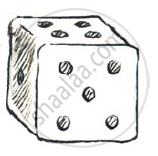Here are two nets to make dice (cubes); the numbers inserted in each square indicate
the number of dots in that box.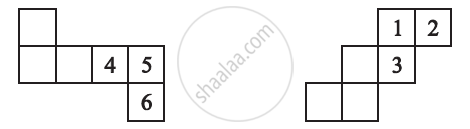Insert suitable numbers in the blanks, remembering that the number on the
opposite faces should total to 7.

Ex. 15.1 | Q 3 | Page 281

Can this be a net for a die?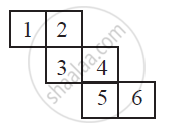Ex. 15.1 | Q 4 | Page 282

Here is an incomplete net for making a cube. Complete it in at least two different ways. Remember that a cube has six faces. How many are there in the net here? (Give two separate diagrams. If you like, you may use a squared sheet for easy manipulation.)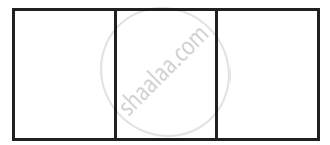Ex. 15.1 | Q 5 | Page 282

Match the nets with appropriate solids:

 a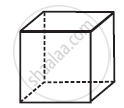1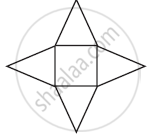b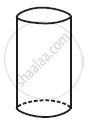2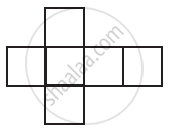c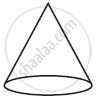3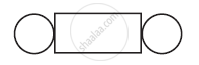d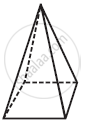4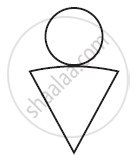#### NCERT solutions for Class 7 Maths Chapter 15 Visualising Solid Shapes Exercise 15.2 [Pages 285 - 286]

Ex. 15.2 | Q 1.1 | Page 285

Use isometric dot paper and make an isometric sketch for of the given shapes: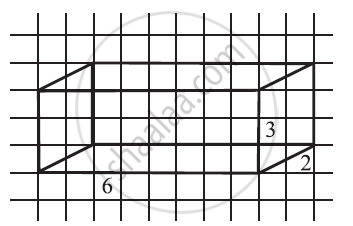Ex. 15.2 | Q 1.2 | Page 285

Use isometric dot paper and make an isometric sketch for of the given shapes: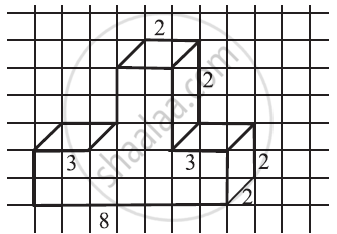Ex. 15.2 | Q 1.3 | Page 285

Use isometric dot paper and make an isometric sketch for of the given shapes: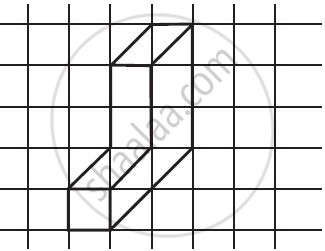Ex. 15.2 | Q 1.4 | Page 285

Use isometric dot paper and make an isometric sketch for of the given shapes: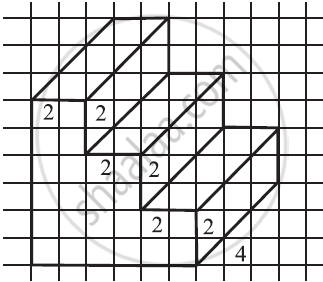Ex. 15.2 | Q 2 | Page 285

The dimensions of a cuboid are 5 cm, 3 cm and 2 cm. Draw three different isometric sketches of this cuboid

Ex. 15.2 | Q 3 | Page 285

Three cubes each with 2 cm edge are placed side by side to form a cuboid. Sketch an oblique or isometric sketch of this cuboid.

Ex. 15.2 | Q 4 | Page 285

Make an oblique sketch for each one of the given isometric shapes: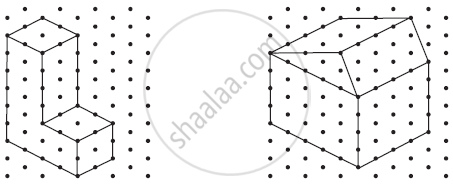Ex. 15.2 | Q 5.1 | Page 286

Give an oblique sketch of the following:

1) A cuboid of dimensions 5 cm, 3 cm and 2 cm. (Is your sketch unique?)

2) A cube with an edge 4 cm long.

Ex. 15.2 | Q 5.2 | Page 286

Give an isometric sketch for the following:

1) A cuboid of dimensions 5 cm, 3 cm and 2 cm. (Is your sketch unique?)

2) A cube with an edge 4 cm long.

#### NCERT solutions for Class 7 Maths Chapter 15 Visualising Solid Shapes Exercise 15.3 [Page 288]

Ex. 15.3 | Q 1.1 | Page 288

What cross-sections do you get when you give a vertical cut and horizontal cut to the given solids a brick.

Ex. 15.3 | Q 1.2 | Page 288

What cross-sections do you get when you give a vertical cut and horizontal cut to the given solids a round apple.

Ex. 15.3 | Q 1.3 | Page 288

What cross-sections do you get when you give a vertical cut and horizontal cut to the given solids a die.

Ex. 15.3 | Q 1.4 | Page 288

What cross-sections do you get when you give a vertical cut and horizontal cut to the given solids a circular pipe.

Ex. 15.3 | Q 1.5 | Page 288

What cross-sections do you get when you give a vertical cut and horizontal cut to the given solids a ice cream cone.

#### NCERT solutions for Class 7 Maths Chapter 15 Visualising Solid Shapes Exercise 15.4 [Pages 289 - 290]

Ex. 15.4 | Q 1 | Page 289

A bulb is kept burning just right above the following solids. Name the shape of the shadows obtained in each case. Attempt to give a rough sketch of the shadow. (You may try to experiment first and then answer these questions).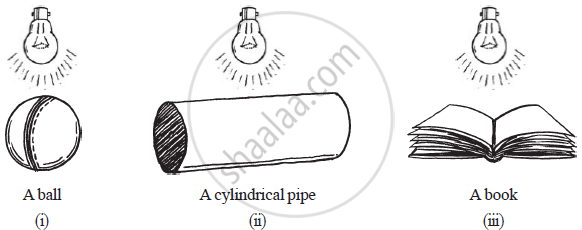Ex. 15.4 | Q 2 | Page 289

Here are the shadows of some 3−D objects, when seen under the lamp of an overhead projector. Identify the solids (s) that match each shadow. (There may be multiple answers for these!)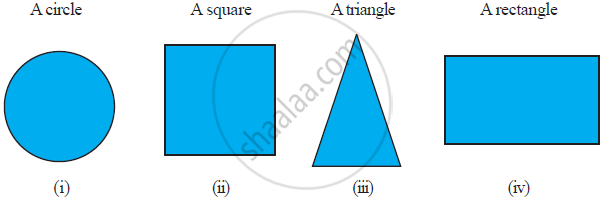Ex. 15.4 | Q 3 | Page 290

Examine if the following are true statements:

1) The cube can cast a shadow in the shape of a rectangle.

2) The cube can cast a shadow in the shape of a hexagon.

## Chapter 15: Visualising Solid Shapes

Ex. 15.1Ex. 15.2Ex. 15.3Ex. 15.4## NCERT solutions for Class 7 Maths chapter 15 - Visualising Solid Shapes

NCERT solutions for Class 7 Maths chapter 15 (Visualising Solid Shapes) include all questions with solution and detail explanation. This will clear students doubts about any question and improve application skills while preparing for board exams. The detailed, step-by-step solutions will help you understand the concepts better and clear your confusions, if any. Shaalaa.com has the CBSE Class 7 Maths solutions in a manner that help students grasp basic concepts better and faster.

Further, we at Shaalaa.com provide such solutions so that students can prepare for written exams. NCERT textbook solutions can be a core help for self-study and acts as a perfect self-help guidance for students.

Concepts covered in Class 7 Maths chapter 15 Visualising Solid Shapes are Introduction of Plane Figures and Solid Shapes, Concept of Faces, Edges and Vertices, Nets for Building 3-d Shapes, Drawing Solids on a Flat Surface - Oblique Sketches, Drawing Solids on a Flat Surface - Isometric Sketches, Drawing Solids on a Flat Surface - Visualising Solid Objects, Viewing Different Sections of a Solid - Another Way is by Shadow Play.

Using NCERT Class 7 solutions Visualising Solid Shapes exercise by students are an easy way to prepare for the exams, as they involve solutions arranged chapter-wise also page wise. The questions involved in NCERT Solutions are important questions that can be asked in the final exam. Maximum students of CBSE Class 7 prefer NCERT Textbook Solutions to score more in exam.

Get the free view of chapter 15 Visualising Solid Shapes Class 7 extra questions for Class 7 Maths and can use Shaalaa.com to keep it handy for your exam preparation